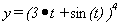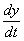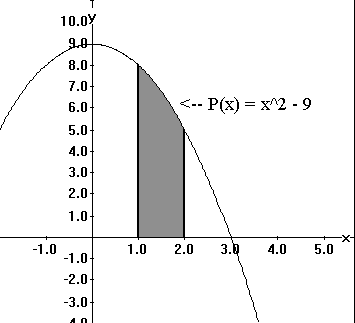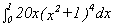MATH 110               BACKGOUNDS REALITY CHECK           M. FLASHMAN

1. Suppose. Find.

2.

Ans.

3. Use calculus to find the maximum value of g(x) = x 3 - 5 x 2 + 2 for x in the interval [-2,3].

Ans.

5. Suppose f is a function with f '(x) = 12x + 4 and f (2) = 5. Find f(0).

6.

Ans. f (0) =

7. Find the area of the region in the plane enclosed by the X-axis, the lines X=1, X=2, and the graph of the function P(x) = 9 -x 2.

8.Ans.

9. Evaluate the integral:10.

Ans.